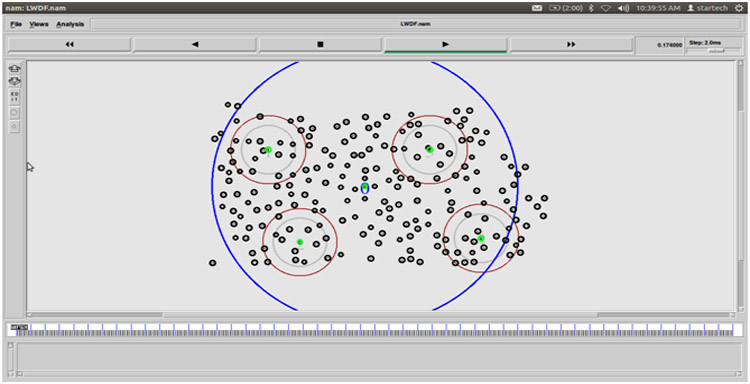﻿ What is Shortest path?Back

## What is Shortest path?SHORTEST PATH

An algorithm that is designed essentially to find a path of minimum length between two specified vertices of a connected weighted graph

·         Initialize the array smallest Weight so that smallest Weight[u] = weights[vertex, u].

·         Set smallest Weight[vertex] = 0.

·         Find the vertex, v, that is closest to vertex for which the shortest path has not been determined.

·         Mark v as the (next) vertex for which the smallest weight is found.

·         For each vertex w in G, such that the shortest path from vertex to w has not been determined and an edge (v, w) exists, if the weight of the path to w via v is smaller than its current weight, update the weight of w to the weight of v + the weight of the edge (v, w).

NS2 CODE FOR SHORTEST PATH ROUTING

DIJIKSTRA’S ROUTING ALGORITHM